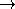## Kinetic::CertificateGenerator

### Definition

This functor allows you to create certificate objects of some type. The models of this ``concept'' take some set of arguments which depend on the certificate being computed (for example three points for a two dimensional orientation) followed by either one or two instances of the Kinetic::Simulator::Time concept. The functions either return a Certificate or the corresponding value at the current time (if only a time value rather than an interval is passed).

### Operations

 Certificate a ( Args , Time begin , Time end ) Return a Certifate object for the corresponding certificate. CGAL::Sign a ( Args , Time t ) Compute the sign of the function at lim0 t+. This can be used to evaluate predicates at the current moment.

### Has Models

All over the place.

### See Also

Kinetic::KineticKernel

### Example

Here you see how to use both functions on an orientation predicate.

```KineticKernel::Point_2 a,b,c;
Simulator::Handle sh;
KineticKernek kk;

KineticKernel::Orientation_2 o2= kk.orientation_2_object();

KineticKernel::Certificate c= o2(a,b,c, sh->current_time(), sh->end_time());
if (c.will_fail()) {
std::cout << "Certificate will fail" << std::endl;
}
// Compute the sign immediately following the current time
CGAL::Sign sn= o2(a,c,b, sh->current_time());
CGAL_postcondition(sn==CGAL::NEGATIVE);

```